On December 1st 2016 I gave a talk in the KGRC Research Seminar.

Abstract: Let $x$ be a real of sufficiently high Turing degree, let $\kappa_x$ be the least inaccessible cardinal in $L[x]$ and let $G$ be $Col(\omega, {<}\kappa_x)$-generic over $L[x]$. Then Woodin has shown that $\operatorname{HOD}^{L[x,G]}$ is a core model, together with a fragment of its own iteration strategy.

Our plan is to extend this result to mice which have finitely many Woodin cardinals. We will introduce a direct limit system of mice due to Grigor Sargsyan and sketch a scenario to show the following result. Let $n \geq 1$ and let $x$ again be a real of sufficiently high Turing degree. Let $\kappa_x$ be the least inaccessible strong cutpoint cardinal of $M_n(x)$ such that $\kappa_x$ is a limit of strong cutpoint cardinals in $M_n(x)$ and let $g$ be $Col(\omega, {<}\kappa_x)$-generic over $M_n(x)$. Then $\operatorname{HOD}^{M_n(x,g)}$ is again a core model, together with a fragment of its own iteration strategy.

This is joint work in progress with Grigor Sargsyan.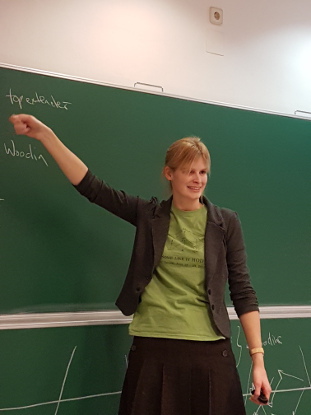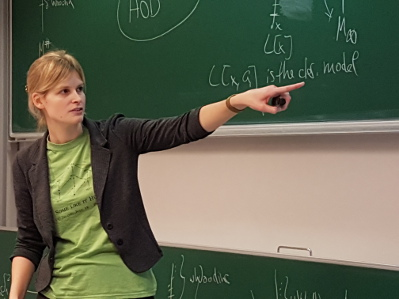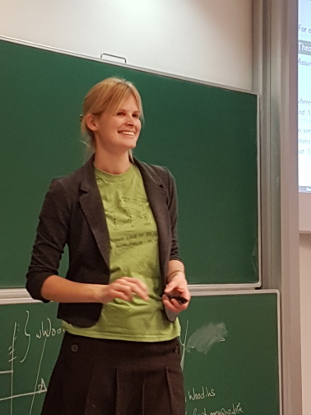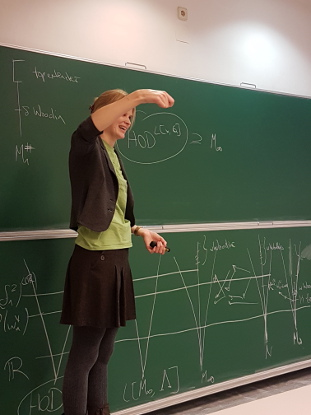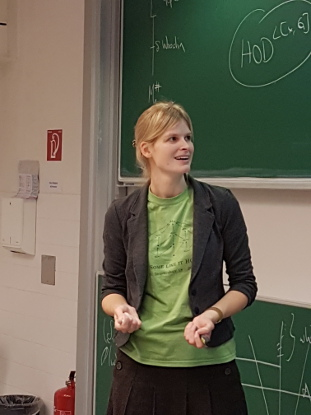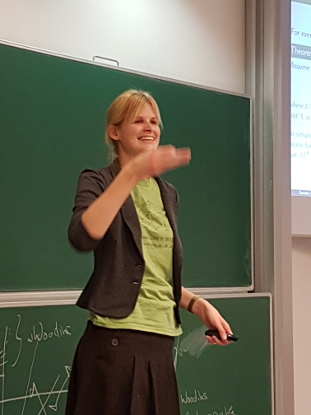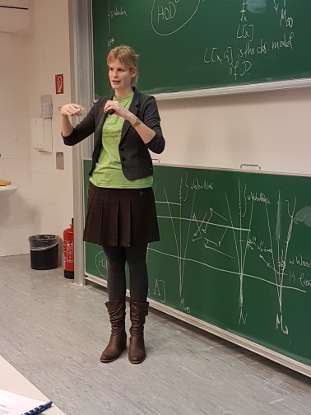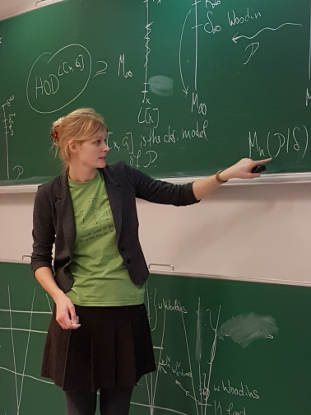Many thanks to Richard again for the great pictures!